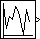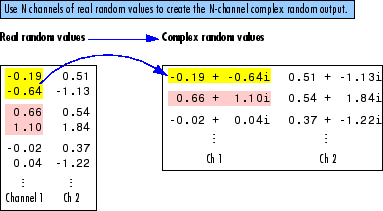# Random Source

Generate randomly distributed values

•Libraries:
DSP System Toolbox / Sources

## Description

The Random Source block generates a frame of `M` values drawn from a uniform or Gaussian pseudorandom distribution. Specify `M` in the Samples per frame parameter.

## Ports

### Output

expand all

Signal of random values with uniform or Gaussian (normal) distribution.

Data Types: `single` | `double`
Complex Number Support: Yes

## Parameters

expand all

The distribution from which to draw the random values, `Uniform` or `Gaussian`.

When you set the Source type parameter to `Uniform`, the output samples are drawn from a uniform distribution whose minimum and maximum values are specified by the Minimum and Maximum parameters, respectively. All values in this range are equally likely to be selected. A length-N vector specified for one or both of these parameters generates an N-channel output (M-by-N matrix) containing a unique random distribution in each channel.

For example, specify

• Minimum = ```[0 0 -3 -3]```

• Maximum = ```[10 10 20 20]```

to generate a four-channel output whose first and second columns contain random values in the range [0, 10], and whose third and fourth columns contain random values in the range [-3, 20]. When you specify only one of the Minimum and Maximum parameters as a vector, the block scalar expands the other parameter so it is the same length as the vector.

When you set the Source type parameter to `Gaussian`, you must also set the Method parameter, which determines the method by which the block computes the output.

The method by which the block computes the Gaussian random values:

• `Ziggurat` — Produces Gaussian random values by using the ziggurat method. This method is equivalent to the ```Legacy MATLAB version 5.0 normal generator``` method.

• `Sum of uniform values` — Produces Gaussian random values by adding and scaling uniformly distributed random signals based on the central limit theorem. This theorem states that the probability distribution of the sum of a sufficiently high number of random variables approaches the Gaussian distribution. You must set the Number of uniform values to sum parameter, which determines the number of uniformly distributed random numbers to sum to produce a single Gaussian random value.

For both settings of the Method parameter, the output samples are drawn from the normal distribution defined by the Mean and Variance parameters. A length-N vector specified for one or both of the Mean and Variance parameters generates an N-channel output (M-by-N frame matrix) containing a distinct random distribution in each column. When you specify only one of these parameters as a vector, the block scalar expands the other parameter so it is the same length as the vector.

#### Dependencies

To enable this parameter, set Source type to `Gaussian`. For more information, see Source type.

The number of uniformly distributed random values to sum to compute a single number in a Gaussian random distribution.

#### Dependencies

To enable this parameter, set Source type to `Gaussian` and Method to `Sum of uniform values`. For more information, see Source type.

The minimum value in the uniform distribution specified as a finite scalar or vector.

Tunable: Yes

#### Dependencies

To enable this parameter, set Source type to `Uniform`.

#### Limitations

Tunable (Simulink) in Simulation mode only.

The maximum value in the uniform distribution specified as a finite scalar or vector.

Tunable: Yes

#### Dependencies

To enable this parameter, set Source type to `Uniform`.

#### Limitations

Tunable (Simulink) in Simulation mode only.

The mean of the Gaussian (normal) distribution specified as a finite scalar or vector.

Tunable: Yes

#### Dependencies

To enable this parameter, set Source type to `Gaussian`.

#### Limitations

Tunable (Simulink) in Simulation mode only.

The variance of the Gaussian (normal) distribution.

#### Dependencies

To enable this parameter, set Source type to `Gaussian`.

#### Limitations

Tunable (Simulink) in Simulation mode only.

The Repeatability parameter determines if the block outputs the same signal each time you run the simulation. You can set the parameter to one of the following options:

• `Repeatable` — Outputs the same signal each time you run the simulation. The first time you run the simulation, the block randomly selects an initial seed. The block reuses these same initial seeds every time you rerun the simulation.

• `Specify seed` — Outputs the same signal each time you run the simulation. Every time you run the simulation, the block uses the initial seeds specified in the Initial seed parameter. Also see Initial seed.

• `Not repeatable` — Does not output the same signal each time you run the simulation. Every time you run the simulation, the block randomly selects an initial seed.

The initial seed(s) to use for the random number generator specified as a finite scalar or vector. The generator produces an identical sequence of pseudorandom numbers each time it is executed with a particular initial seed.

To specify the N initial seeds for an N-channel real-valued output, set the Complexity parameter to `Real` and provide one of the following in the Initial seed parameter:

• Length-N vector of initial seeds — Uses each vector element as an initial seed for the corresponding channel in the N-channel output.

• Single scalar — Uses the scalar to generate N random values as the seeds for the N-channel output.

To specify the initial seeds for an N-channel complex-valued output, set the Complexity parameter to `Complex` and provide one of the following in the Initial seed parameter:

• Length-N vector of initial seeds — Uses each vector element as an initial seed for generating N channels of real random values. The block uses pairs of adjacent values in each of these channels as the real and imaginary components of the final output, as illustrated in the following figure.

• Single scalar — Uses the scalar to generate N random values as the seeds for generating N channels of real random values. The block uses pairs of adjacent values in each of these channels as the real and imaginary components of the final output, as illustrated in the following figure.Tunable: Yes

#### Dependencies

To enable this parameter, set Repeatability to `Specify seed`.

#### Limitations

Tunable (Simulink) in Simulation mode only.

When you select this check box, the block inherits the sample mode, sample time, output data type, complexity, and signal dimensions of the signal from the downstream block. When you select this check box, the Sample mode, Sample time, Samples per frame, Output data type, and Complexity parameters are disabled.

Suppose that you want to back propagate a 1-D vector. The output of the Random Source block is a 1-D vector of length M, where length M is inherited from the downstream block. When the Minimum, Maximum, Mean, or Variance parameter specifies N channels, the 1-D vector output contains M/N samples from each channel. An error occurs in this case when M is not an integer multiple of `N`.

Suppose that you want to back propagate a M-by-N signal. When N>`1`, your signal has N channels. When N = `1`, your signal has M channels. The value of the Minimum, Maximum, Mean, or Variance parameter can be a scalar or a vector of length equal to the number of channels. You can specify these parameters as either row or column vectors, except when the signal is a row vector. In this case, the Minimum, Maximum, Mean, or Variance parameter must also be specified as a row vector.

The sample mode, specified as `Continuous` or `Discrete`.

When you set Sample mode to `Discrete`, the Sample time parameter value, Ts, specifies the random sequence sample period. In this mode, the block generates the number of samples specified by the Samples per frame parameter value, M, and outputs this frame with a period of MTs.

When you set Sample mode to `Continuous`, the block is configured for continuous-time operation, and the Sample time and Samples per frame parameters are disabled. Note that many DSP System Toolbox™ blocks do not accept continuous-time inputs.

#### Dependencies

To enable this parameter, clear the Inherit output port attributes check box.

The sample period, Ts, of the random output sequence when the Sample mode is `Discrete`, specified as a positive, finite, scalar. The output frame period is MTs.

#### Dependencies

To enable this parameter, clear the Inherit output port attributes check box and set Sample mode to `Discrete`.

The number of samples, M, in each output frame, specified as a positive integer. The output frame period is MTs.

#### Dependencies

To enable this parameter, clear the Inherit output port attributes check box and set Sample mode to `Discrete`.

The data type of the output, specified as single-precision or double-precision.

#### Dependencies

To enable this parameter, clear the Inherit output port attributes check box.

The complexity of the output, specified as `Real` or `Complex`. These settings control all channels of the output, so real and complex data cannot be combined in the same output. For complex output with a `Uniform` distribution, the real and imaginary components in each channel are both drawn from the same uniform random distribution, defined by the Minimum and Maximum parameters for that channel.

For complex output with a `Gaussian` distribution, the real and imaginary components in each channel are drawn from normal distributions with different means. In this case, the Mean parameter for each channel should specify a complex value; the real component of the Mean parameter specifies the mean of the real components in the channel, while the imaginary component specifies the mean of the imaginary components in the channel. When either the real or imaginary component is omitted from the Mean parameter, a default value of 0 is used for the mean of that component.

For example, a Mean parameter setting of `[5+2i 0.5 3i]` generates a three-channel output with the following means.

 Channel 1 mean real = 5 imaginary = 2 Channel 2 mean real = 0.5 imaginary = 0 Channel 3 mean real = 0 imaginary = 3

For complex output, the Variance parameter, σ2, specifies the total variance for each output channel. This is the sum of the variances of the real and imaginary components in that channel.

`${\sigma }^{2}={\sigma }_{\mathrm{Re}}^{2}+{\sigma }_{\mathrm{Im}}^{2}$`

The specified variance is equally divided between the real and imaginary components, so that

`$\begin{array}{c}{\sigma }_{\mathrm{Re}}^{2}=\frac{{\sigma }^{2}}{2}\\ {\sigma }_{\mathrm{Im}}^{2}=\frac{{\sigma }^{2}}{2}\end{array}$`

#### Dependencies

To enable this parameter, clear the Inherit output port attributes check box.

## Block Characteristics

 Data Types `double` | `single` Direct Feedthrough `no` Multidimensional Signals `no` Variable-Size Signals `no` Zero-Crossing Detection `no`

## Version History

Introduced before R2006a